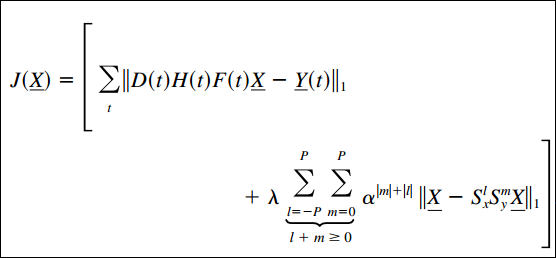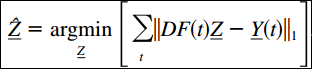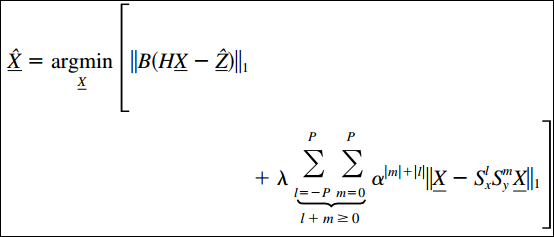# Week 5: Advances and Challenges in Super-Resolution

I am returning to reading literature about image Super Resolution and have taken a look at Sina Farsiu, Dirk Robinson, Michael Elad, and Peyman Milanfar’s work into Super resolution.  On Milanfar’s page, there are 4 papers regarding work in this subject and has covered both still and video applications of their work.  In the future I will look into these different papers and over time try and port/create an implementation of this outside of their MATLAB code which they have used for prototyping

Introduction

Super resolution is categorized as an inverse problem.  This is because we are trying to reverse the output of the camera system/capture system to recreate the original real life/source representation of in this case the image.

Since the dawn of SR the idea was as follows.$\underline{Y(t)}=M(t)\underline{X(t)}+V(t)$

Where$\underline{Y(t)}$ is defined as the time varying output of the image system,$M(t)$ represents the imaging system (qualities such as blurring or fidelity of the hardware)$\underline{X(t)}$ represents the real life scene, and$V(t)$ represents the noise that could have been introduced (for example imperfections in the lense).  Note: the underline means it is a vector.

With this in mind we need to “invert” this equation such that the answer$\underline{X(t)}$ is the new output.  In order to determine the best solution we must take into account a cost function.

The cost function is used to gauge the fidelity of the output solution.  The classic, and now proven to be ineffective/costly, solution uses the least squares method to detemrine the difference from the estimate solution to the real life scene.$\underline{\hat{X}}=argmin(\underline{X})||\underline{Y}-M\underline{X}||_2^2+\lambda \rho(\underline{X})$

Here the$\lambda$ and$\rho(\underline{X})$ represent a scaling factor and penalty function which is used to limit the number of solutions to this high dimension linear algebra equation.  This is not only done in the interest of computation time but also in the interest of ruling out many outlier solutions which may just introduce large amounts of noise.  To speed up the calculation matrices are used for the high dimension calculations.

Although the idea is simple modern data implementations of these cost functions often use machine learning and neural networks to train the penalty function.  Milanfar and his team’s main contribution will be offering a computationally efficient and more accurate version of this cost function which uses 1D ($L_1$) instead of 2D ($L_2$) to measure the error.

Describing M the Model Matrix

In general the model matrix is described as follows.$M=DAHF$

M: Model matrix
D/A: Sampling effects of the sensor
H: Point spread function
F: Scalar to preserve the intensity of the image, and image motion

Note: The point spread function is essentially the impulse response of an imaging system.  Like in audio processing applications, this impulse response is the inherent change that is caused by the hardware itself.  For audio it could be an unintentional LP or HP of the signal or noise.  Parameters of the imaging system will be stored in D, A, and H.

Note 2: Motion prediction in F is hard/impossible to do accurately in systems due to the random nature of subjects (such as live organisms) movement.  Here as proof of concept (due to simplicity and the simplification in calculation) Milanfar and others used the translational model.  It is important to note that one of the major simplifications this model enables is the commutative nature of the model Matrix’s arguments above.

Milanfar’s Work on the Cost Function$J(\underline{X})$ is the cost function developed by Milanfar and those that worked with him.  As mentioned before they use the$L_1$ norm to calculate error instead of$L_2$.  Their work has shown that$L_1$ exhibits robustness to data outliers which cause noise in the$L_2$ domain.  Here M has been replaced by the description of the model matrix above.

The penalty function is the other point of interest.$\lambda$ remains a scaling factor.  However, they implement the translational model here.

The summation is across “l pixels” in the x direction and “m pixels” in the y direction.  S terms serve as the operators for shifting and alpha is the scaling factor.

Calculation of the high resolution output X

The calculation now can be calculated in two steps.

1. Produce a blurred high resolution of the image$\underline{\hat{Z}}$
2. Deblur and denoise the image.step 1: this is summed over multiple frames “t”step 2

Here B represents a diagonal matrix of weights equal to the number of measurements made at a specific element.  This ensures that those elements that were not sampled (at all or as much) are not considered as heavily.  Ensuring that the interpolation will be done from actual samples from the camera sensor.

Sources:
Farsiu, S., Robinson, D., Elad, M., & Milanfar, P. (2004). Advances and challenges in super-resolution. International Journal of Imaging Systems and Technology, 14(2), 47-57. doi:10.1002/ima.20007